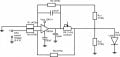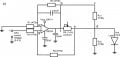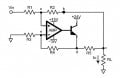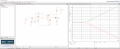# How to build the voltage controlled current source based on modified Hawland current pump with FET?

#### DX400

Joined Oct 26, 2017
12
Hi,

As noted in the subject, I have a problem with building of the voltage controlled current source with obligatory grounded load. The input control voltage is in the range from 0 to 5 V, the output voltage is up to 20 V and output current on the load up to 150 mA. The load is some semiconductor device( or material), the (light-emitting)diode for example. Other very important requirements are that the load must be grounded, the control voltage on input and the voltages on the output should be measured with respect to the one common point(ground).

I have already used the modified Howland current pump. The scheme is quite good for output current of 25 mA, but the suitable heat sink was required to protect the OpAmp. Also I have used the unipolar voltage supply of the OpAmp to obtain the 20 V of output. The another benefit of this scheme is that the output current does not depend on the load resistance.How to modify the Howland current pump with the grounded load for output current up to about 200 mA (150 required with about 10 -40 mA in reserve)?

I have found the example of such modification in the Ref. https://www.edn.com/design/analog/4...nimize-errors-in-grounded-load-current-source. The Howland current pump based, with n-channel FET switch.I have tried this scheme with OpAmp LM324 and FET IRLB8743, using resistors R1=R2=R3=100 kOhm, R4=104 kOhm, and obtain the output current up to 200 mA by varying of R5 (pot of 5 kOhm) controlling resistor.
But as I found from the circuit calculations, presented in the Ref, the output current is dependent on the load resistance. Hence, this current source is quite bad.
It is possible to improve the scheme to resolve this problem and to obtain voltage controlled current source with grounded load for output current up to about 150-200 mA?

#### DX400

Joined Oct 26, 2017
12

#### crutschow

Joined Mar 14, 2008
30,147
the output current is dependent on the load resistance.
That's only true in general, but not if the resistor values are properly selected.

Let R1=R3 and R2=R4 (post #2 circuit).

If R5 is much smaller than R2, then the output current gain is basically the differential gain (R2/R1) divided by R5 or R2/(R1R5), independent of the load impedance.

To maximize the available output voltage, use a small value for R5 so that its drop is <1V at the maximum output current.

Then set the differential gain so that a 5V input gives a voltage across R5 equal to the maximum desired current through RL (hint: the differential gain can be set to less than one).

Note, that in your first circuit, the op amp's minus power pin must be connected to ground.

•DX400

#### DX400

Joined Oct 26, 2017
12
Thank you very much for the answers.

But, how to check the value of the current on load (Iout) in the second circuit?

In first circuit there are two resistors Rc1 and Rc2. The voltage on the R5 (control) is equal to voltage difference Uc1 and Uc2, being measured on the Rc1 and Rc2 with respect to the common (ground) point. So, the current on load is equal U5(voltage on R5)/R5?

But how to control the current value in case of circuit #2? Only by means of gain value?

#### crutschow

Joined Mar 14, 2008
30,147
In first circuit there are two resistors Rc1 and Rc2. The voltage on the R5 (control) is equal to voltage difference Uc1 and Uc2, being measured on the Rc1 and Rc2 with respect to the common (ground) point.
I don't understand what Rc1 and Rc2 are supposed to do, and they seem unnecessary, which is why they aren't in the second circuit.
So, the current on load is equal U5(voltage on R5)/R5?
Depends on what you mean by "voltage on R5".
If it's the voltage to ground, no.
If you mean the voltage across R5's terminals, then yes.
That voltage is determined by Vin times the circuit differential gain.
But how to control the current value in case of circuit #2? Only by means of gain value?
Yes, you change the current gain, either from the value of R5, or the differential gain (R2/R1) of the circuit.

Make sense?

Last edited:
•DX400

#### DX400

Joined Oct 26, 2017
12
Thank you very much for the answers. I have made a correction to my sketches to make a better guide for an eye.So,
In my main task I must use the PC controlled board for voltage measurements. All ADC channels of my board are dependent by one common ground pin named "AGND". Hence all measurements must be perforemed between some point of circuit and the ground pin.
For example, on sketch #1 the voltage on load is the voltage between point "d" and ground.
The points b, c and f are equivalent. The points e and d are also equivalent. So voltage difference between URc1(measured between point "c" and ground) and URc2(between point "d" and ground) gives the voltage on R5 (voltage across R5's terminals) between f and e, which allows me to obtain current on the load.
Resistors Rc1 and Rc2 allows to mesure current.

#### DX400

Joined Oct 26, 2017
12
don't understand what Rc1 and Rc2 are supposed to do
Resistors Rc1 and Rc2 allow me to mesure current on load when circuit is created, and the voltage is input voltage is varied.
I am unable to measure voltage between f and e points, only between some point and the ground.

Yes, you change the current gain, either from the value of R5, or the differential gain (R2/R1) of the circuit.
I understand it. The resistor R5 allows me to set current range of the circuit.
But my question is about how to measure current on load in case of scheme #2?
Lets assume, the circuit #2 is created and the input voltage varies. The voltage on load is the voltage between point "c" and ground.
Here I am unable to put some resistors to mesure voltage across R5. So, the only way to measure current on load is to use the transfer relation, which postulate
If R5 is much smaller than R2, then the output current gain is basically the differential gain (R2/R1) divided by R5 or R2/(R1R5), independent of the load impedance.
So, Iout=R2/(R1R5). Right?

Another question is whether It is necessary to put some voltage follower between point c and b to reduce some current distortions, which may be caused by resistor R2? The (+) i from point c and the output to point b.

#### crutschow

Joined Mar 14, 2008
30,147
But my question is about how to measure current on load in case of scheme #2?
The voltage across Rc1 and Rc2 can't directly tell you the current unless you also measure the voltage across the load.
You can then calculate the voltage drop across R5 to determine the current.
(I don't see a reason for the resistors. Just measure the op amp output voltage and the load voltage to get R5's voltage.)

Otherwise just add a small resistor in series with the load to ground, and measure the voltage drop across the resistor.
For example, a 10Ω resistor will give a voltage of 10mV per mA.
So, Iout=R2/(R1R5). Right?
No. That's the current gain.

You need to look at your equations and see if the units make sense.
For example, dividing a resistance by a resistance, as you show, does not give a value for current, it gives a value of current gain (amps per volt).
To get a current, it's voltage times the gain, or Iout = Vin * R2/(R1R5).
Another question is whether It is necessary to put some voltage follower between point c and b to reduce some current distortions, which may be caused by resistor R2? The (+) i from point c and the output to point b.
You could add a resistor equal to R5 to help balance the circuit, but if R5 is much smaller than R2, it will have only a very small effect on the voltage to current accuracy.

Same for adding a voltage follower.

•DX400

#### DX400

Joined Oct 26, 2017
12
There is always this approach.
I have tried this schematics, but it doesn't work for me. I had used MOSFET as a transistor. May be I have made something wrong.

#### crutschow

Joined Mar 14, 2008
30,147
Do you still want the current to be controlled by a 0-5V input, with 0V giving zero current?
If so, the circuit in post #9 doesn't readily work for that.

The Howland circuit is probably the best circuit for that requirement with a grounded load.

•DX400

#### DX400

Joined Oct 26, 2017
12
Do you still want the current to be controlled by a 0-5V input, with 0V giving zero current?
Yes I do.

If so, the circuit in post #9 doesn't readily work for that.
I have already understand it some time ago, trying to build the current sources like in post #9. So I came to Hawland based scheme.

The Howland circuit is probably the best circuit for that requirement with a grounded load.
I agree. And I hope the scheme #2 is the solution for me.

Joined Mar 10, 2018
4,057
You needed the output to supply 150 mA, so conventional Howland you would have
to use a power OpAmp.

Thanks crutschow for catching my error on input range, blindness is a constant
challenge for me.

I fooled around with modifying simple current source and got the following results in sim -I think if you get dual RRIO OpAmp you could make this work. The initial
flat portion of curve I think due to violation of LM324 input CM range, it
does not include + rail which is a problem for this approach. RRIO should
fix that.

Regards, Dana.

Joined Mar 10, 2018
4,057
Last edited:

#### crutschow

Joined Mar 14, 2008
30,147
I agree. And I hope the scheme #2 is the solution for me.
Yes, I think that's the best solution for your problem.

I haven't posted a simulation of the circuit since this is Homework Help, and I want you to finish the design by yourself.But the simulation does work very well, with less than 0.05% change in current with a load change from zero ohms to the maximum resistance it can drive.

Last edited: